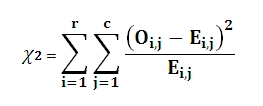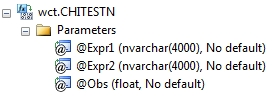# SQL Server CHISQ.TEST function

CHITESTN

Updated: 28 February 2011

Note: This documentation is for the SQL2008 (and later) version of this XLeratorDB function, it is not compatible with SQL Server 2005.

Use the aggregate function CHITESTN to calculate the Pearson chi-square test for independence on normalized tables. CHITESTN returns the value from the chi-square (χ2) distribution for the chi-square statistic and the appropriate degrees of freedom.  Calculate the chi-square statistic (χ2) directly using the CHISQN or the CHISQN_q function.

The chi-square statistic is calculated by finding the difference between each observed and theoretical frequency for each possible outcome, squaring them, dividing each by the theoretical frequency, and taking the sum of the results. A second important part of determining the test statistic is to define the degrees of freedom of the test: this is essentially the number of squares errors involving the observed frequencies adjusted for the effect of using some of those observations to define the expected frequencies.

Given the test statistic and the degrees of freedom, the test value is returned by the regularized gamma function Q(a, x) where:

a             is the degrees of freedom divided by 2
x              is χ2 statistic divided by 2

CHITESTN automatically calculates the expected results and the degrees of freedom.

The value of the test statistic isWhere
r              is the number of rows
c              is the number of columns
O             is the Observed result
E              is the Expected result
SyntaxArguments
@Expr1
the name or number of the rows.
@Expr2
the name or number of the columns.
@Obs
the observed value. @Obs is an expression of type float or of a type that can be implicitly converted to float.
Return Types
float
Remarks
·         CHITESTN is designed for normalized tables. For de-normalized tables, use the scalar function CHITEST.
·         CHITESTN automatically calculates the expected values.
·         CHITESTN = CHIDIST(χ2, df), where df = (r-1)(c-1), r>1, c>1.
·         Use the CHISQN function to calculate the test statistic.
·         CHITESTN is an aggregate function and follows the same conventions as all other aggregate functions in SQL Server.
·         If you have previously used the CHITESTN scalar function, the CHITESTN aggregate has a different syntax. The CHITESTN scalar function is no longer available in XLeratorDB/statistics2008, though you can still use the scalar CHITESTN _q.

Examples
In this hypothetical situation, we want to determine if there is an association between population density and the preference for a sport from among baseball, football, and basketball. We will use the CHITESTN function to perform the chi-square test.
SELECT wct.CHITESTN(
sport       --@Expr1
,locale     --@Expr2
,result     --@obs
) as CHITEST
FROM (VALUES
('Baseball', 'Rural', 60),
('Baseball', 'Suburban', 43),
('Baseball', 'Urban', 35),
('Football', 'Rural', 52),
('Football', 'Suburban', 48),
('Football', 'Urban', 28)
) n(sport, locale, result)

This produces the following result
CHITEST
----------------------
0.000162912223138266

(1 row(s) affected)

What if the data had not been normalized? We could use that following SQL to take de-normalized data (the way it might be in a spreadsheet) and turn it into normalized data.
SELECT wct.CHITESTN(n.sport, x.locale, x.result) as CHITEST
FROM (VALUES
('Baseball', 60, 43, 35),
('Football', 52, 48, 28)
) n(sport, rural, suburban, urban)
CROSS APPLY(VALUES
('rural', rural),
('suburban', suburban),
('urban',urban)
) x(locale, result)

This produces the following result.
CHITEST
----------------------
0.000162912223138266

(1 row(s) affected)

In this example, the de-normalized table contains another column, region. We will calculate the chi-square test for each region.
SELECT n.region,
wct.CHITESTN(n.sport, x.locale, x.result) as CHITEST
FROM (VALUES
('Midwest','Baseball',27,38,36),
('Midwest','Football',43,29,36),
('Northeast','Baseball',39,46,42),
('Northeast','Football',35,30,41),
('Southeast','Baseball',31,36,33),
('Southeast','Football',31,24,40),
('Southwest','Baseball',44,19,34),
('Southwest','Football',41,29,36),
('West','Baseball',43,33,30),
('West','Football',30,36,30)
) n(region, sport, urban, rural, suburban)
CROSS APPLY(VALUES
('rural', rural),
('suburban', suburban),
('urban',urban)
) x(locale, result)
GROUP BY region

This produces the following result.
region                   CHITEST
--------- ----------------------
Midwest       0.145577930808542
Northeast      0.577611987659069
Southeast      0.374156016716296
Southwest     0.0186392153050728
West           0.725711264565586

(5 row(s) affected)

### SupportCopyright 2008-2023 Westclintech LLC         Privacy Policy        Terms of Service